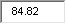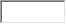/
/
/
Question that passes through the inductor? 1 Hz (b) What the capacitance of
Not my Question
Flag Content

# Question : Question that passes through the inductor? 1 Hz (b) What the capacitance of

Question

that passes through the inductor?

1Hz

(b) What is the capacitance of a capacitor that has the same

reactance at this frequency?

2F

(c) The frequency is tripled, so that the reactances of the

inductor and capacitor are no longer equal. What is the new

reactance of the inductor?

(d) What is the new reactance of the capacitor?